Test: Kirchhoff’s Laws & Network Solutions

# Test: Kirchhoff’s Laws & Network Solutions

Test Description

## 10 Questions MCQ Test Basic Electrical Technology | Test: Kirchhoff’s Laws & Network Solutions

Test: Kirchhoff’s Laws & Network Solutions for Electrical Engineering (EE) 2022 is part of Basic Electrical Technology preparation. The Test: Kirchhoff’s Laws & Network Solutions questions and answers have been prepared according to the Electrical Engineering (EE) exam syllabus.The Test: Kirchhoff’s Laws & Network Solutions MCQs are made for Electrical Engineering (EE) 2022 Exam. Find important definitions, questions, notes, meanings, examples, exercises, MCQs and online tests for Test: Kirchhoff’s Laws & Network Solutions below.
Solutions of Test: Kirchhoff’s Laws & Network Solutions questions in English are available as part of our Basic Electrical Technology for Electrical Engineering (EE) & Test: Kirchhoff’s Laws & Network Solutions solutions in Hindi for Basic Electrical Technology course. Download more important topics, notes, lectures and mock test series for Electrical Engineering (EE) Exam by signing up for free. Attempt Test: Kirchhoff’s Laws & Network Solutions | 10 questions in 10 minutes | Mock test for Electrical Engineering (EE) preparation | Free important questions MCQ to study Basic Electrical Technology for Electrical Engineering (EE) Exam | Download free PDF with solutions
 1 Crore+ students have signed up on EduRev. Have you?
Test: Kirchhoff’s Laws & Network Solutions - Question 1

### Find the value of I1, I2 and I3.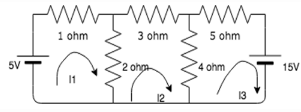Detailed Solution for Test: Kirchhoff’s Laws & Network Solutions - Question 1

Using the matrix method:
Matrix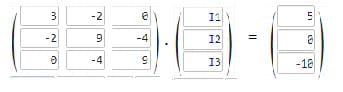Solving this matrix equation, we get I1=1.5A,

I2=-0.188A

I3=-1.19A.

Test: Kirchhoff’s Laws & Network Solutions - Question 2

### Find the value of V, if the value of I3= 0A.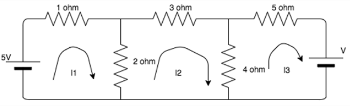Detailed Solution for Test: Kirchhoff’s Laws & Network Solutions - Question 2

5-3I1+2I2=0, 9I2-2I1=0, -4I2+V=0
On solving,V=1.739V.

Test: Kirchhoff’s Laws & Network Solutions - Question 3

### Find the value of R if the power in the circuit is 1000W.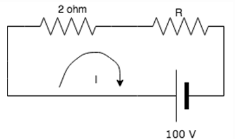Detailed Solution for Test: Kirchhoff’s Laws & Network Solutions - Question 3

To find the value of I:
VI=P =>100I=1000 => I=10A.
Voltage across the 2 ohm resistor= 20V.
Voltage across the R resistor= 100-20= 80V.
R=V/I => R=80/10= 8ohm.

Test: Kirchhoff’s Laws & Network Solutions - Question 4

Find the current in the 4 ohm resistor.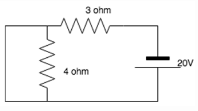Detailed Solution for Test: Kirchhoff’s Laws & Network Solutions - Question 4

The 4 ohm resistor gets shorted since current always prefers the low resistance path. All the current flows to the branch which is connected in parallel to the 4 ohm branch, hence no current flows in the 4 ohm resistance.

Test: Kirchhoff’s Laws & Network Solutions - Question 5

Nodal analysis is generally used to determine_______

Detailed Solution for Test: Kirchhoff’s Laws & Network Solutions - Question 5

Nodal analysis uses Kirchhoff’s Current Law to find all the node voltages. Hence it is a method used to determine voltage.

Test: Kirchhoff’s Laws & Network Solutions - Question 6

Mesh analysis is generally used to determine_________

Detailed Solution for Test: Kirchhoff’s Laws & Network Solutions - Question 6

Mesh analysis uses Kirchhoff’s Voltage Law to find all the mesh currents. Hence it is a method used to determine current.

Test: Kirchhoff’s Laws & Network Solutions - Question 7

What is the current in the circuit?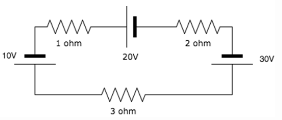Detailed Solution for Test: Kirchhoff’s Laws & Network Solutions - Question 7

If we move in the clockwise direction, we get the total voltage to be equal to: -10-20+30= 0V. Since I=V/R= 0/4=0, I=0A.

Test: Kirchhoff’s Laws & Network Solutions - Question 8

Does the 15A source have any effect on the circuit?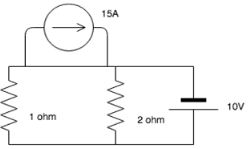Detailed Solution for Test: Kirchhoff’s Laws & Network Solutions - Question 8

The 15A current source has a lower resistance path associated with it and hence it keeps moving in that particular loop. It does not leave that loop and enter the circuit, hence the circuit is not affected by it.

Test: Kirchhoff’s Laws & Network Solutions - Question 9

KVL is associated with____________

Detailed Solution for Test: Kirchhoff’s Laws & Network Solutions - Question 9

KVL employs mesh analysis to find the different mesh currents by finding the IR products in each mesh.

Test: Kirchhoff’s Laws & Network Solutions - Question 10

Kirchhoff's laws are valid for

Detailed Solution for Test: Kirchhoff’s Laws & Network Solutions - Question 10

KCL employs nodal analysis to find the different node voltages by finding the value if current in each branch.

## Basic Electrical Technology

57 docs|59 tests
 Use Code STAYHOME200 and get INR 200 additional OFF Use Coupon Code
Information about Test: Kirchhoff’s Laws & Network Solutions Page
In this test you can find the Exam questions for Test: Kirchhoff’s Laws & Network Solutions solved & explained in the simplest way possible. Besides giving Questions and answers for Test: Kirchhoff’s Laws & Network Solutions, EduRev gives you an ample number of Online tests for practice

57 docs|59 tests